# 10 Linear Equations in One Variable

### Learning Objectives

In this section you will:

• Solve equations in one variable algebraically.
• Solve a rational equation.
• Find a linear equation.
• Given the equations of two lines, determine whether their graphs are parallel or perpendicular.
• Write the equation of a line parallel or perpendicular to a given line.

Caroline is a full-time college student planning a spring break vacation. To earn enough money for the trip, she has taken a part-time job at the local bank that pays $15.00/hr, and she opened a savings account with an initial deposit of$400 on January 15. She arranged for direct deposit of her payroll checks. If spring break begins March 20 and the trip will cost approximately $2,500, how many hours will she have to work to earn enough to pay for her vacation? If she can only work 4 hours per day, how many days per week will she have to work? How many weeks will it take? In this section, we will investigate problems like this and others, which generate graphs like the line in (Figure).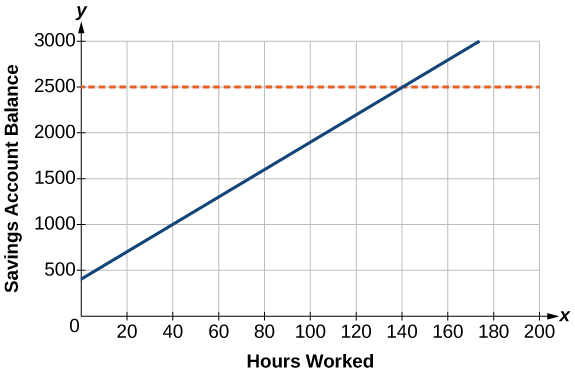Figure 1. ### Solving Linear Equations in One Variable A linear equation is an equation of a straight line, written in one variable. The only power of the variable is 1. Linear equations in one variable may take the form$\,ax+b=0\,$and are solved using basic algebraic operations. We begin by classifying linear equations in one variable as one of three types: identity, conditional, or inconsistent. An identity equation is true for all values of the variable. Here is an example of an identity equation. $3x=2x+x$ The solution set consists of all values that make the equation true. For this equation, the solution set is all real numbers because any real number substituted for$\,x\,$will make the equation true. A conditional equation is true for only some values of the variable. For example, if we are to solve the equation$\,5x+2=3x-6,$we have the following: $\begin{array}{ccc}\hfill 5x+2& =& 3x-6\hfill \\ \hfill 2x& =& -8\hfill \\ \hfill x& =& -4\hfill \end{array}$ The solution set consists of one number:$\,\left\{-4\right\}.\,$It is the only solution and, therefore, we have solved a conditional equation. An inconsistent equation results in a false statement. For example, if we are to solve$\,5x-15=5\left(x-4\right),$we have the following: $\begin{array}{cccc}\hfill 5x-15& =& 5x-20\hfill & \\ \hfill 5x-15-5x& =& 5x-20-5x\hfill & \phantom{\rule{2em}{0ex}}\text{Subtract }5x\text{ from both sides}.\hfill \\ \hfill -15& \ne & -20\hfill & \phantom{\rule{2em}{0ex}}\text{False statement}\hfill \end{array}$ Indeed,$\,-15\ne \,-20.\,$There is no solution because this is an inconsistent equation. Solving linear equations in one variable involves the fundamental properties of equality and basic algebraic operations. A brief review of those operations follows. ### Linear Equation in One Variable A linear equation in one variable can be written in the form $ax+b=0$ where a and b are real numbers,$\,a\ne 0.$ ### How To Given a linear equation in one variable, use algebra to solve it. The following steps are used to manipulate an equation and isolate the unknown variable, so that the last line reads$\,x=\text{_________,}$if x is the unknown. There is no set order, as the steps used depend on what is given: 1. We may add, subtract, multiply, or divide an equation by a number or an expression as long as we do the same thing to both sides of the equal sign. Note that we cannot divide by zero. 2. Apply the distributive property as needed:$\,a\left(b+c\right)=ab+ac.$ 3. Isolate the variable on one side of the equation. 4. When the variable is multiplied by a coefficient in the final stage, multiply both sides of the equation by the reciprocal of the coefficient. ### Solving an Equation in One Variable Solve the following equation:$\,2x+7=19.$ [reveal-answer q=”fs-id780975″]Show Solution[/reveal-answer] [hidden-answer a=”fs-id780975″]This equation can be written in the form$\,ax+b=0\,$by subtracting$\,19\,$from both sides. However, we may proceed to solve the equation in its original form by performing algebraic operations. $\begin{array}{cccc}\hfill 2x+7& =& 19\hfill & \\ \hfill 2x& =& 12\hfill & \phantom{\rule{2em}{0ex}}\text{Subtract 7 from both sides}\text{.}\hfill \\ \hfill x& =& 6\hfill & \phantom{\rule{2em}{0ex}}\text{Multiply both sides by }\frac{1}{2}\text{ or divide by 2}\text{.}\hfill \end{array}$ The solution is 6 [/hidden-answer] ### Try It Solve the linear equation in one variable:$\,2x+1=-9.$ [reveal-answer q=”fs-id1237626″]Show Solution[/reveal-answer] [hidden-answer a=”fs-id1237626″] $x=-5$ [/hidden-answer] ### Solving an Equation Algebraically When the Variable Appears on Both Sides Solve the following equation:$\,4\left(x-3\right)+12=15-5\left(x+6\right).$ [reveal-answer q=”fs-id1943089″]Show Solution[/reveal-answer] [hidden-answer a=”fs-id1943089″] Apply standard algebraic properties. $\begin{array}{cccc}\hfill 4\left(x-3\right)+12& =& 15-5\left(x+6\right)\hfill & \\ 4x-12+12\hfill & =& 15-5x-30\hfill & \phantom{\rule{2em}{0ex}}\text{Apply the distributive property}\text{.}\hfill \\ \hfill 4x& =& -15-5x\hfill & \phantom{\rule{2em}{0ex}}\text{Combine like terms}.\hfill \\ \hfill 9x& =& -15\hfill & \phantom{\rule{2em}{0ex}}\text{Place }x-\text{terms on one side and simplify}.\hfill \\ \hfill x& =& -\frac{15}{9}\hfill & \phantom{\rule{2em}{0ex}}\text{Multiply both sides by }\frac{1}{9}\text{, the reciprocal of 9}.\hfill \\ \hfill x& =& -\frac{5}{3}\hfill & \end{array}$[/hidden-answer] #### Analysis This problem requires the distributive property to be applied twice, and then the properties of algebra are used to reach the final line,$\,x=-\frac{5}{3}.$ ### Try It Solve the equation in one variable:$\,-2\left(3x-1\right)+x=14-x.$ [reveal-answer q=”fs-id3094701″]Show Solution[/reveal-answer] [hidden-answer a=”fs-id3094701″] $x=-3$ [/hidden-answer] ### Solving a Rational Equation In this section, we look at rational equations that, after some manipulation, result in a linear equation. If an equation contains at least one rational expression, it is a considered a rational equation. Recall that a rational number is the ratio of two numbers, such as$\,\frac{2}{3}\,$or$\,\frac{7}{2}.\,$A rational expression is the ratio, or quotient, of two polynomials. Here are three examples. $\frac{x+1}{{x}^{2}-4},\,\frac{1}{x-3},\,\text{or}\,\frac{4}{{x}^{2}+x-2}$ Rational equations have a variable in the denominator in at least one of the terms. Our goal is to perform algebraic operations so that the variables appear in the numerator. In fact, we will eliminate all denominators by multiplying both sides of the equation by the least common denominator (LCD). Finding the LCD is identifying an expression that contains the highest power of all of the factors in all of the denominators. We do this because when the equation is multiplied by the LCD, the common factors in the LCD and in each denominator will equal one and will cancel out. ### Solving a Rational Equation Solve the rational equation:$\,\frac{7}{2x}-\frac{5}{3x}=\frac{22}{3}.$ [reveal-answer q=”fs-id2708670″]Show Solution[/reveal-answer] [hidden-answer a=”fs-id2708670″] We have three denominators;$\,2x,3x,$and 3. The LCD must contain$\,2x,3x,$and 3. An LCD of$\,6x\,$contains all three denominators. In other words, each denominator can be divided evenly into the LCD. Next, multiply both sides of the equation by the LCD$\,6x.$ $\begin{array}{cccc}\hfill \left(6x\right)\left[\frac{7}{2x}-\frac{5}{3x}\right]& =& \left[\frac{22}{3}\right]\left(6x\right)\hfill & \\ \hfill \left(6x\right)\left(\frac{7}{2x}\right)-\left(6x\right)\left(\frac{5}{3x}\right)& =& \left(\frac{22}{3}\right)\left(6x\right)\hfill & \phantom{\rule{2em}{0ex}}\text{Use the distributive property}.\hfill \\ \hfill \left(\overline{)6x}\right)\left(\frac{7}{\overline{)2x}}\right)-\left(\overline{)6x}\right)\left(\frac{5}{\overline{)3x}}\right)& =& \left(\frac{22}{\overline{)3}}\right)\left(\overline{)6}x\right)\hfill & \phantom{\rule{2em}{0ex}}\text{Cancel out the common factors}.\hfill \\ \hfill 3\left(7\right)-2\left(5\right)& =& 22\left(2x\right)\hfill & \phantom{\rule{2em}{0ex}}\text{Multiply remaining factors by each numerator}.\hfill \\ \hfill 21-10& =& 44x\hfill & \\ \hfill 11& =& 44x\hfill & \\ \hfill \frac{11}{44}& =& x\hfill & \\ \hfill \frac{1}{4}& =& x\hfill & \end{array}$[/hidden-answer] A common mistake made when solving rational equations involves finding the LCD when one of the denominators is a binomial—two terms added or subtracted—such as$\,\left(x+1\right).\,$Always consider a binomial as an individual factor—the terms cannot be separated. For example, suppose a problem has three terms and the denominators are$\,x,$$x-1,$and$\,3x-3.\,$First, factor all denominators. We then have$\,x,$$\left(x-1\right),$and$\,3\left(x-1\right)\,$as the denominators. (Note the parentheses placed around the second denominator.) Only the last two denominators have a common factor of$\,\left(x-1\right).\,$The$\,x\,$in the first denominator is separate from the$\,x\,$in the$\,\left(x-1\right)\,$denominators. An effective way to remember this is to write factored and binomial denominators in parentheses, and consider each parentheses as a separate unit or a separate factor. The LCD in this instance is found by multiplying together the$\,x,$one factor of$\,\left(x-1\right),$and the 3. Thus, the LCD is the following: $x\left(x-1\right)3=3x\left(x-1\right)$ So, both sides of the equation would be multiplied by$\,3x\left(x-1\right).\,$Leave the LCD in factored form, as this makes it easier to see how each denominator in the problem cancels out. Another example is a problem with two denominators, such as$\,x\,$and$\,{x}^{2}+2x.\,$Once the second denominator is factored as$\,{x}^{2}+2x=x\left(x+2\right),$there is a common factor of x in both denominators and the LCD is$\,x\left(x+2\right).$ Sometimes we have a rational equation in the form of a proportion; that is, when one fraction equals another fraction and there are no other terms in the equation. $\frac{a}{b}=\frac{c}{d}$ We can use another method of solving the equation without finding the LCD: cross-multiplication. We multiply terms by crossing over the equal sign.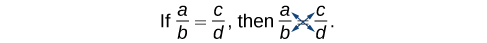Multiply$\,a\left(d\right)\,$and$\,b\left(c\right),$which results in$\,ad=bc.$ Any solution that makes a denominator in the original expression equal zero must be excluded from the possibilities. ### Rational Equations A rational equation contains at least one rational expression where the variable appears in at least one of the denominators. ### How To Given a rational equation, solve it. 1. Factor all denominators in the equation. 2. Find and exclude values that set each denominator equal to zero. 3. Find the LCD. 4. Multiply the whole equation by the LCD. If the LCD is correct, there will be no denominators left. 5. Solve the remaining equation. 6. Make sure to check solutions back in the original equations to avoid a solution producing zero in a denominator ### Solving a Rational Equation without Factoring Solve the following rational equation: $\frac{2}{x}-\frac{3}{2}=\frac{7}{2x}$ [reveal-answer q=”fs-id3141796″]Show Solution[/reveal-answer] [hidden-answer a=”fs-id3141796″] We have three denominators:$\,x,$$2,$and$\,2x.\,$No factoring is required. The product of the first two denominators is equal to the third denominator, so, the LCD is$\,2x.\,$Only one value is excluded from a solution set, 0. Next, multiply the whole equation (both sides of the equal sign) by$\,2x.$ $\begin{array}{cccc}\hfill 2x\left[\frac{2}{x}-\frac{3}{2}\right]& =& \left[\frac{7}{2x}\right]2x& \\ \hfill 2\overline{)x}\left(\frac{2}{\overline{)x}}\right)-\overline{)2}x\left(\frac{3}{\overline{)2}}\right)& =& \left(\frac{7}{\overline{)2x}}\right)\overline{)2x}\hfill & \phantom{\rule{2em}{0ex}}\text{Distribute }2x\text{.}\hfill \\ \hfill 2\left(2\right)-3x& =& 7\hfill & \phantom{\rule{2em}{0ex}}\text{Denominators cancel out}.\hfill \\ \hfill 4-3x& =& 7\hfill & \\ \hfill -3x& =& 3\hfill & \\ \hfill x& =& -1\hfill & \\ & & \text{or}\phantom{\rule{0.3em}{0ex}}\left\{-1\right\}\hfill & \end{array}$ The proposed solution is −1, which is not an excluded value, so the solution set contains one number,$\,x=-1,$or$\,\left\{-1\right\}\,$written in set notation.[/hidden-answer] ### Try It Solve the rational equation:$\,\frac{2}{3x}=\frac{1}{4}-\frac{1}{6x}.$ [reveal-answer q=”fs-id2931856″]Show Solution[/reveal-answer] [hidden-answer a=”fs-id2931856″] $x=\frac{10}{3}$ [/hidden-answer] ### Solving a Rational Equation by Factoring the Denominator Solve the following rational equation:$\,\frac{1}{x}=\frac{1}{10}-\frac{3}{4x}.$ [reveal-answer q=”fs-id2436750″]Show Solution[/reveal-answer] [hidden-answer a=”fs-id2436750″] First find the common denominator. The three denominators in factored form are$\,x,10=2\cdot 5,$and$\,4x=2\cdot 2\cdot x.\,$The smallest expression that is divisible by each one of the denominators is$\,20x.\,$Only$\,x=0\,$is an excluded value. Multiply the whole equation by$\,20x.$ $\begin{array}{ccc}\hfill 20x\left(\frac{1}{x}\right)& =& \left(\frac{1}{10}-\frac{3}{4x}\right)20x\hfill \\ \hfill 20& =& 2x-15\hfill \\ \hfill 35& =& 2x\hfill \\ \hfill \frac{35}{2}& =& x\hfill \end{array}$ The solution is$\,\frac{35}{2}.$[/hidden-answer] ### Try It Solve the rational equation:$\,-\frac{5}{2x}+\frac{3}{4x}=-\frac{7}{4}.$ [reveal-answer q=”fs-id1149728″]Show Solution[/reveal-answer] [hidden-answer a=”fs-id1149728″] $x=1$ [/hidden-answer] ### Solving Rational Equations with a Binomial in the Denominator Solve the following rational equations and state the excluded values: 1. $\frac{3}{x-6}=\frac{5}{x}$ 2. $\frac{x}{x-3}=\frac{5}{x-3}-\frac{1}{2}$ 3. $\frac{x}{x-2}=\frac{5}{x-2}-\frac{1}{2}$ [reveal-answer q=”fs-id1996554″]Show Solution[/reveal-answer] [hidden-answer a=”fs-id1996554″] 1. The denominators$\,x\,$and$\,x-6\,$have nothing in common. Therefore, the LCD is the product$\,x\left(x-6\right).\,$However, for this problem, we can cross-multiply. $\begin{array}{cccc}\hfill \frac{3}{x-6}& =& \frac{5}{x}\hfill & \\ \hfill 3x& =& 5\left(x-6\right)\hfill & \phantom{\rule{2em}{0ex}}\text{Distribute}\text{.}\hfill \\ \hfill 3x& =& 5x-30\hfill & \\ \hfill -2x& =& -30\hfill & \\ \hfill x& =& 15\hfill & \end{array}$ The solution is 15. The excluded values are $6$ and $0.$ 2. The LCD is$\,2\left(x-3\right).\,$Multiply both sides of the equation by$\,2\left(x-3\right).$ $\begin{array}{ccc}\hfill 2\left(x-3\right)\left[\frac{x}{x-3}\right]& =& \left[\frac{5}{x-3}-\frac{1}{2}\right]2\left(x-3\right)\hfill \\ \hfill \frac{2\overline{)\left(x-3\right)}x}{\overline{)x-3}}& =& \frac{2\overline{)\left(x-3\right)}5}{\overline{)x-3}}-\frac{\overline{)2}\left(x-3\right)}{\overline{)2}}\hfill \\ \hfill 2x& =& 10-\left(x-3\right)\hfill \\ \hfill 2x& =& 10-x+3\hfill \\ \hfill 2x& =& 13-x\hfill \\ \hfill 3x& =& 13\hfill \\ \hfill x& =& \frac{13}{3}\hfill \end{array}$ The solution is$\,\frac{13}{3}.\,$The excluded value is $3.$ 3. The least common denominator is$\,2\left(x-2\right).\,$Multiply both sides of the equation by$\,x\left(x-2\right).$ $\begin{array}{ccc}\hfill 2\left(x-2\right)\left[\frac{x}{x-2}\right]& =& \left[\frac{5}{x-2}-\frac{1}{2}\right]2\left(x-2\right)\hfill \\ \hfill 2x& =& 10-\left(x-2\right)\hfill \\ \hfill 2x& =& 12-x\hfill \\ \hfill 3x& =& 12\hfill \\ \hfill x& =& 4\hfill \end{array}$ The solution is 4. The excluded value is $2.$ [/hidden-answer] ### Try It Solve$\,\frac{-3}{2x+1}=\frac{4}{3x+1}.\,$State the excluded values. [reveal-answer q=”fs-id1570666″]Show Solution[/reveal-answer] [hidden-answer a=”fs-id1570666″] $x=-\frac{7}{17}.\,$Excluded values are$\,x=-\frac{1}{2}\,$and$\,x=-\frac{1}{3}.$ [/hidden-answer] ### Solving a Rational Equation with Factored Denominators and Stating Excluded Values Solve the rational equation after factoring the denominators:$\,\frac{2}{x+1}-\frac{1}{x-1}=\frac{2x}{{x}^{2}-1}.\,$State the excluded values. [reveal-answer q=”fs-id2437930″]Show Solution[/reveal-answer] [hidden-answer a=”fs-id2437930″] We must factor the denominator$\,{x}^{2}-1.\,$We recognize this as the difference of squares, and factor it as$\,\left(x-1\right)\left(x+1\right).\,$Thus, the LCD that contains each denominator is$\,\left(x-1\right)\left(x+1\right).\,$Multiply the whole equation by the LCD, cancel out the denominators, and solve the remaining equation. $\begin{array}{ccc}\hfill \left(x-1\right)\left(x+1\right)\left[\frac{2}{x+1}-\frac{1}{x-1}\right]& =& \left[\frac{2x}{\left(x-1\right)\left(x+1\right)}\right]\left(x-1\right)\left(x+1\right)\hfill \\ \hfill 2\left(x-1\right)-1\left(x+1\right)& =& 2x\hfill \\ \hfill 2x-2-x-1& =& 2x\phantom{\rule{2em}{0ex}}\text{Distribute the negative sign}.\hfill \\ \hfill -3-x& =& 0\hfill \\ \hfill -3& =& x\hfill \end{array}$ The solution is $\,-3.\,$The excluded values are $\,1\,$ and $\,-1.$[/hidden-answer] ### Try It Solve the rational equation:$\,\frac{2}{x-2}+\frac{1}{x+1}=\frac{1}{{x}^{2}-x-2}.$ [reveal-answer q=”fs-id1567907″]Show Solution[/reveal-answer] [hidden-answer a=”fs-id1567907″] $x=\frac{1}{3}$ [/hidden-answer] ### Finding a Linear Equation Perhaps the most familiar form of a linear equation is the slope-intercept form, written as$\,y=mx+b,$where$\,m=\text{slope}\,$and$\,b=y\text{−intercept}\text{.}\,$Let us begin with the slope. #### The Slope of a Line The slope of a line refers to the ratio of the vertical change in y over the horizontal change in x between any two points on a line. It indicates the direction in which a line slants as well as its steepness. Slope is sometimes described as rise over run. $m=\frac{{y}_{2}-{y}_{1}}{{x}_{2}-{x}_{1}}$ If the slope is positive, the line slants to the right. If the slope is negative, the line slants to the left. As the slope increases, the line becomes steeper. Some examples are shown in (Figure). The lines indicate the following slopes:$\,m=-3,$$m=2,$and$\,m=\frac{1}{3}.$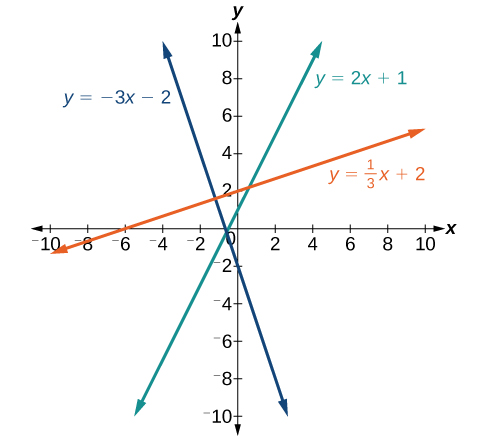Figure 2. ### The Slope of a Line The slope of a line, m, represents the change in y over the change in x. Given two points,$\,\left({x}_{1},{y}_{1}\right)\,$and$\,\left({x}_{2},{y}_{2}\right),$the following formula determines the slope of a line containing these points: $m=\frac{{y}_{2}-{y}_{1}}{{x}_{2}-{x}_{1}}$ ### Finding the Slope of a Line Given Two Points Find the slope of a line that passes through the points$\,\left(2,-1\right)\,$and$\,\left(-5,3\right).$ [reveal-answer q=”fs-id2715022″]Show Solution[/reveal-answer] [hidden-answer a=”fs-id2715022″] We substitute the y-values and the x-values into the formula. $\begin{array}{ccc}\hfill m& =& \frac{3-\left(-1\right)}{-5-2}\hfill \\ & =& \frac{4}{-7}\hfill \\ & =& -\frac{4}{7}\hfill \end{array}$ The slope is$\,-\frac{4}{7}.$[/hidden-answer] #### Analysis It does not matter which point is called$\,\left({x}_{1},{y}_{1}\right)\,$or$\,\left({x}_{2},{y}_{2}\right).\,$As long as we are consistent with the order of the y terms and the order of the x terms in the numerator and denominator, the calculation will yield the same result. ### Try It Find the slope of the line that passes through the points$\,\left(-2,6\right)\,$and$\,\left(1,4\right).$ [reveal-answer q=”fs-id2437070″]Show Solution[/reveal-answer] [hidden-answer a=”fs-id2437070″] $m=-\frac{2}{3}$ [/hidden-answer] ### Identifying the Slope and y-intercept of a Line Given an Equation Identify the slope and y-intercept, given the equation$\,y=-\frac{3}{4}x-4.$ [reveal-answer q=”fs-id1763671″]Show Solution[/reveal-answer] [hidden-answer a=”fs-id1763671″] As the line is in$\,y=mx+b\,$form, the given line has a slope of$\,m=-\frac{3}{4}.\,$The y-intercept is$\,b=-4.$ [/hidden-answer] #### Analysis The y-intercept is the point at which the line crosses the y-axis. On the y-axis,$\,x=0.\,$We can always identify the y-intercept when the line is in slope-intercept form, as it will always equal b. Or, just substitute$\,x=0\,$and solve for y. #### The Point-Slope Formula Given the slope and one point on a line, we can find the equation of the line using the point-slope formula. $y-{y}_{1}=m\left(x-{x}_{1}\right)$ This is an important formula, as it will be used in other areas of college algebra and often in calculus to find the equation of a tangent line. We need only one point and the slope of the line to use the formula. After substituting the slope and the coordinates of one point into the formula, we simplify it and write it in slope-intercept form. ### The Point-Slope Formula Given one point and the slope, the point-slope formula will lead to the equation of a line: $y-{y}_{1}=m\left(x-{x}_{1}\right)$ ### Finding the Equation of a Line Given the Slope and One Point Write the equation of the line with slope$\,m=-3\,$and passing through the point$\,\left(4,8\right).\,$Write the final equation in slope-intercept form. [reveal-answer q=”fs-id1562535″]Show Solution[/reveal-answer] [hidden-answer a=”fs-id1562535″] Using the point-slope formula, substitute$\,-3\,$for m and the point$\,\left(4,8\right)\,$for$\,\left({x}_{1},{y}_{1}\right).$ $\begin{array}{ccc}\hfill y-{y}_{1}& =& m\left(x-{x}_{1}\right)\hfill \\ \hfill y-8& =& -3\left(x-4\right)\hfill \\ \hfill y-8& =& -3x+12\hfill \\ \hfill y& =& -3x+20\hfill \end{array}$[/hidden-answer] #### Analysis Note that any point on the line can be used to find the equation. If done correctly, the same final equation will be obtained. ### Try It Given$\,m=4,$find the equation of the line in slope-intercept form passing through the point$\,\left(2,5\right).$ [reveal-answer q=”fs-id1577661″]Show Solution[/reveal-answer] [hidden-answer a=”fs-id1577661″] $y=4x-3$ [/hidden-answer] ### Finding the Equation of a Line Passing Through Two Given Points Find the equation of the line passing through the points$\,\left(3,4\right)\,$and$\,\left(0,-3\right).\,$Write the final equation in slope-intercept form. [reveal-answer q=”fs-id1920251″]Show Solution[/reveal-answer] [hidden-answer a=”fs-id1920251″] First, we calculate the slope using the slope formula and two points. $\begin{array}{ccc}\hfill m& =& \frac{-3-4}{0-3}\hfill \\ & =& \frac{-7}{-3}\hfill \\ & =& \frac{7}{3}\hfill \end{array}$ Next, we use the point-slope formula with the slope of$\,\frac{7}{3},$and either point. Let’s pick the point$\,\left(3,4\right)\,$for$\,\left({x}_{1},{y}_{1}\right).$ $\begin{array}{ccc}\hfill y-4& =& \frac{7}{3}\left(x-3\right)\hfill \\ \hfill y-4& =& \frac{7}{3}x-7\phantom{\rule{2em}{0ex}}\text{Distribute the }\frac{7}{3}.\hfill \\ \hfill y& =& \frac{7}{3}x-3\hfill \end{array}$ In slope-intercept form, the equation is written as$\,y=\frac{7}{3}x-3.$[/hidden-answer] #### Analysis To prove that either point can be used, let us use the second point$\,\left(0,-3\right)\,$and see if we get the same equation. $\begin{array}{ccc}\hfill y-\left(-3\right)& =& \frac{7}{3}\left(x-0\right)\hfill \\ \hfill y+3& =& \frac{7}{3}x\hfill \\ \hfill y& =& \frac{7}{3}x-3\hfill \end{array}$ We see that the same line will be obtained using either point. This makes sense because we used both points to calculate the slope. #### Standard Form of a Line Another way that we can represent the equation of a line is in standard form. Standard form is given as $Ax+By=C$ where$\,A,$$B,$and$\,C$are integers. The x- and y-terms are on one side of the equal sign and the constant term is on the other side. ### Finding the Equation of a Line and Writing It in Standard Form Find the equation of the line with$\,m=-6\,$and passing through the point$\,\left(\frac{1}{4},-2\right).\,$Write the equation in standard form. [reveal-answer q=”fs-id2753839″]Show Solution[/reveal-answer] [hidden-answer a=”fs-id2753839″] We begin using the point-slope formula. $\begin{array}{ccc}\hfill y-\left(-2\right)& =& -6\left(x-\frac{1}{4}\right)\hfill \\ \hfill y+2& =& -6x+\frac{3}{2}\hfill \end{array}$ From here, we multiply through by 2, as no fractions are permitted in standard form, and then move both variables to the left aside of the equal sign and move the constants to the right. $\begin{array}{ccc}\hfill 2\left(y+2\right)& =& \left(-6x+\frac{3}{2}\right)2\hfill \\ \hfill 2y+4& =& -12x+3\hfill \\ \hfill 12x+2y& =& -1\hfill \end{array}$ This equation is now written in standard form.[/hidden-answer] ### Try It Find the equation of the line in standard form with slope$\,m=-\frac{1}{3}\,$and passing through the point$\,\left(1,\frac{1}{3}\right).$ [reveal-answer q=”fs-id1804908″]Show Solution[/reveal-answer] [hidden-answer a=”fs-id1804908″] $x+3y=2$ [/hidden-answer] #### Vertical and Horizontal Lines The equations of vertical and horizontal lines do not require any of the preceding formulas, although we can use the formulas to prove that the equations are correct. The equation of a vertical line is given as $x=c$ where c is a constant. The slope of a vertical line is undefined, and regardless of the y-value of any point on the line, the x-coordinate of the point will be c. Suppose that we want to find the equation of a line containing the following points:$\,\left(-3,-5\right),\left(-3,1\right),\left(-3,3\right),$and$\,\left(-3,5\right).\,$First, we will find the slope. $m=\frac{5-3}{-3-\left(-3\right)}=\frac{2}{0}$ Zero in the denominator means that the slope is undefined and, therefore, we cannot use the point-slope formula. However, we can plot the points. Notice that all of the x-coordinates are the same and we find a vertical line through$\,x=-3.\,$See (Figure). The equation of a horizontal line is given as $y=c$ where c is a constant. The slope of a horizontal line is zero, and for any x-value of a point on the line, the y-coordinate will be c. Suppose we want to find the equation of a line that contains the following set of points:$\,\left(-2,-2\right),\left(0,-2\right),\left(3,-2\right),$and$\,\left(5,-2\right).$We can use the point-slope formula. First, we find the slope using any two points on the line. $\begin{array}{ccc}\hfill m& =& \frac{-2-\left(-2\right)}{0-\left(-2\right)}\hfill \\ & =& \frac{0}{2}\hfill \\ & =& 0\hfill \end{array}$ Use any point for$\,\left({x}_{1},{y}_{1}\right)\,$in the formula, or use the y-intercept. $\begin{array}{ccc}\hfill y-\left(-2\right)& =& 0\left(x-3\right)\hfill \\ \hfill y+2& =& 0\hfill \\ \hfill y& =& -2\hfill \end{array}$ The graph is a horizontal line through$\,y=-2.\,$Notice that all of the y-coordinates are the same. See (Figure).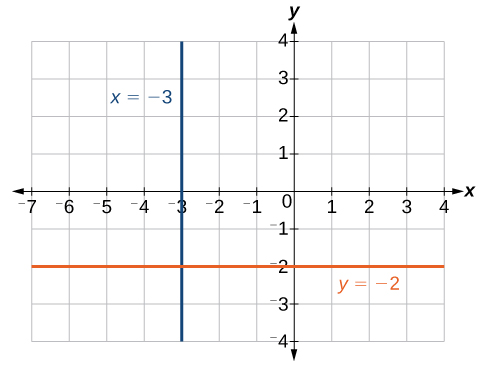Figure 3. The line x = −3 is a vertical line. The line y = −2 is a horizontal line. ### Finding the Equation of a Line Passing Through the Given Points Find the equation of the line passing through the given points:$\,\left(1,-3\right)\,$and$\,\left(1,4\right).$ [reveal-answer q=”fs-id2764547″]Show Solution[/reveal-answer] [hidden-answer a=”fs-id2764547″] The x-coordinate of both points is 1. Therefore, we have a vertical line,$\,x=1.$ [/hidden-answer] ### Try It Find the equation of the line passing through$\,\left(-5,2\right)\,$and$\,\left(2,2\right).$ [reveal-answer q=”fs-id1551241″]Show Solution[/reveal-answer] [hidden-answer a=”fs-id1551241″] Horizontal line:$\,y=2$ [/hidden-answer] ### Determining Whether Graphs of Lines are Parallel or Perpendicular Parallel lines have the same slope and different y-intercepts. Lines that are parallel to each other will never intersect. For example, (Figure) shows the graphs of various lines with the same slope,$\,m=2.$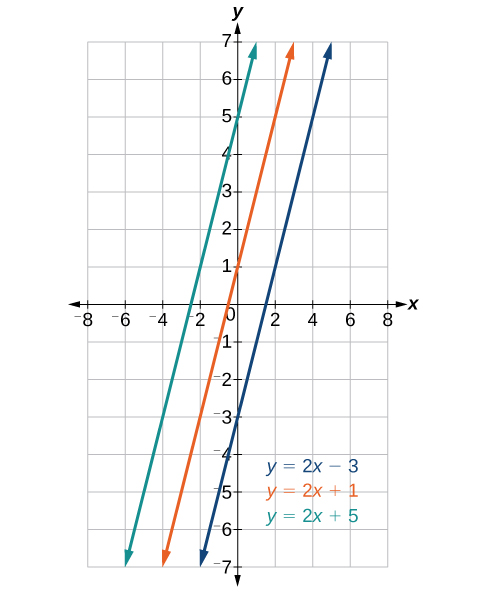Figure 4. Parallel lines All of the lines shown in the graph are parallel because they have the same slope and different y-intercepts. Lines that are perpendicular intersect to form a$\,90°$-angle. The slope of one line is the negative reciprocal of the other. We can show that two lines are perpendicular if the product of the two slopes is$\,-1:{m}_{1}\cdot {m}_{2}=-1.\,$For example, (Figure) shows the graph of two perpendicular lines. One line has a slope of 3; the other line has a slope of$\,-\frac{1}{3}.$ $\begin{array}{ccc}\hfill {m}_{1}\cdot {m}_{2}& =& -1\hfill \\ \hfill 3\cdot \left(-\frac{1}{3}\right)& =& -1\hfill \end{array}$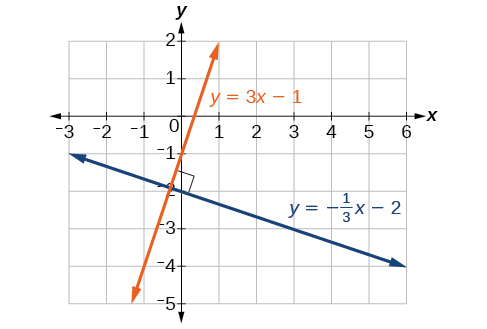Figure 5. Perpendicular lines ### Graphing Two Equations, and Determining Whether the Lines are Parallel, Perpendicular, or Neither Graph the equations of the given lines, and state whether they are parallel, perpendicular, or neither:$\,3y=-4x+3\,$and$\,3x-4y=8.$ [reveal-answer q=”fs-id2931413″]Show Solution[/reveal-answer] [hidden-answer a=”fs-id2931413″] The first thing we want to do is rewrite the equations so that both equations are in slope-intercept form. First equation: $\begin{array}{ccc}\hfill 3y& =& -4x+3\hfill \\ \hfill y& =& -\frac{4}{3}x+1\hfill \end{array}$ Second equation: $\begin{array}{ccc}\hfill 3x-4y& =& 8\hfill \\ \hfill -4y& =& -3x+8\hfill \\ \hfill y& =& \frac{3}{4}x-2\hfill \end{array}$ See the graph of both lines in (Figure)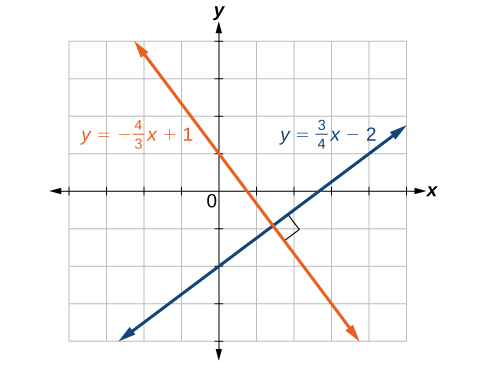Figure 6. From the graph, we can see that the lines appear perpendicular, but we must compare the slopes. $\begin{array}{ccc}\hfill {m}_{1}& =& -\frac{4}{3}\hfill \\ \hfill {m}_{2}& =& \frac{3}{4}\hfill \\ \hfill {m}_{1}\cdot {m}_{2}& =& \left(-\frac{4}{3}\right)\left(\frac{3}{4}\right)=-1\hfill \end{array}$ The slopes are negative reciprocals of each other, confirming that the lines are perpendicular.[/hidden-answer] ### Try It Graph the two lines and determine whether they are parallel, perpendicular, or neither:$\,2y-x=10\,$and$\,2y=x+4.$ [reveal-answer q=”fs-id2403402″]Show Solution[/reveal-answer] [hidden-answer a=”fs-id2403402″] Parallel lines: equations are written in slope-intercept form.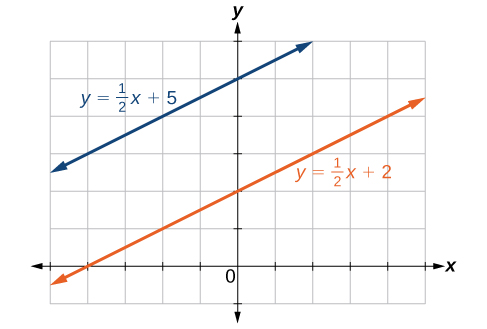[/hidden-answer] ### Writing the Equations of Lines Parallel or Perpendicular to a Given Line As we have learned, determining whether two lines are parallel or perpendicular is a matter of finding the slopes. To write the equation of a line parallel or perpendicular to another line, we follow the same principles as we do for finding the equation of any line. After finding the slope, use the point-slope formula to write the equation of the new line. ### How To Given an equation for a line, write the equation of a line parallel or perpendicular to it. 1. Find the slope of the given line. The easiest way to do this is to write the equation in slope-intercept form. 2. Use the slope and the given point with the point-slope formula. 3. Simplify the line to slope-intercept form and compare the equation to the given line. ### Writing the Equation of a Line Parallel to a Given Line Passing Through a Given Point Write the equation of line parallel to a$\,5x+3y=1\,$and passing through the point$\,\left(3,5\right).$ [reveal-answer q=”fs-id1531339″]Show Solution[/reveal-answer] [hidden-answer a=”fs-id1531339″] First, we will write the equation in slope-intercept form to find the slope. $\begin{array}{ccc}\hfill 5x+3y& =& 1\hfill \\ \hfill 3y& =& 5x+1\hfill \\ \hfill y& =& -\frac{5}{3}x+\frac{1}{3}\hfill \end{array}$ The slope is$\,m=-\frac{5}{3}.\,$The y-intercept is$\,\frac{1}{3},$but that really does not enter into our problem, as the only thing we need for two lines to be parallel is the same slope. The one exception is that if the y-intercepts are the same, then the two lines are the same line. The next step is to use this slope and the given point with the point-slope formula. $\begin{array}{ccc}\hfill y-5& =& -\frac{5}{3}\left(x-3\right)\hfill \\ \hfill y-5& =& -\frac{5}{3}x+5\hfill \\ \hfill y& =& -\frac{5}{3}x+10\hfill \end{array}$ The equation of the line is$\,y=-\frac{5}{3}x+10.\,$See (Figure).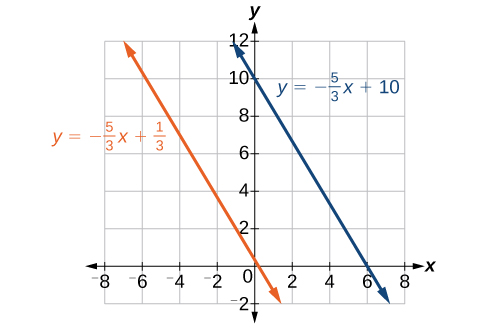Figure 7. [/hidden-answer] ### Try It Find the equation of the line parallel to$\,5x=7+y\,$and passing through the point$\,\left(-1,-2\right).$ [reveal-answer q=”fs-id2769990″]Show Solution[/reveal-answer] [hidden-answer a=”fs-id2769990″] $y=5x+3$ [/hidden-answer] ### Finding the Equation of a Line Perpendicular to a Given Line Passing Through a Given Point Find the equation of the line perpendicular to$\,5x-3y+4=0\,\,\left(-4,1\right).$ [reveal-answer q=”fs-id1790303″]Show Solution[/reveal-answer] [hidden-answer a=”fs-id1790303″] The first step is to write the equation in slope-intercept form. $\begin{array}{ccc}\hfill 5x-3y+4& =& 0\hfill \\ \hfill -3y& =& -5x-4\hfill \\ \hfill y& =& \frac{5}{3}x+\frac{4}{3}\hfill \end{array}$ We see that the slope is$\,m=\frac{5}{3}.\,$This means that the slope of the line perpendicular to the given line is the negative reciprocal, or $-\frac{3}{5}.\,$Next, we use the point-slope formula with this new slope and the given point. $\begin{array}{ccc}\hfill y-1& =& -\frac{3}{5}\left(x-\left(-4\right)\right)\hfill \\ \hfill y-1& =& -\frac{3}{5}x-\frac{12}{5}\hfill \\ \hfill y& =& -\frac{3}{5}x-\frac{12}{5}+\frac{5}{5}\hfill \\ \hfill y& =& -\frac{3}{5}x-\frac{7}{5}\hfill \end{array}$[/hidden-answer] Access these online resources for additional instruction and practice with linear equations. ### Key Concepts • We can solve linear equations in one variable in the form$\,ax+b=0\,$using standard algebraic properties. See (Figure) and (Figure). • A rational expression is a quotient of two polynomials. We use the LCD to clear the fractions from an equation. See (Figure) and (Figure). • All solutions to a rational equation should be verified within the original equation to avoid an undefined term, or zero in the denominator. See (Figure) and (Figure). • Given two points, we can find the slope of a line using the slope formula. See (Figure). • We can identify the slope and y-intercept of an equation in slope-intercept form. See (Figure). • We can find the equation of a line given the slope and a point. See (Figure). • We can also find the equation of a line given two points. Find the slope and use the point-slope formula. See (Figure). • The standard form of a line has no fractions. See (Figure). • Horizontal lines have a slope of zero and are defined as$\,y=c,$where c is a constant. • Vertical lines have an undefined slope (zero in the denominator), and are defined as$\,x=c,$where c is a constant. See (Figure). • Parallel lines have the same slope and different y-intercepts. See (Figure). • Perpendicular lines have slopes that are negative reciprocals of each other unless one is horizontal and the other is vertical. See (Figure). ### Section Exercises #### Verbal What does it mean when we say that two lines are parallel? [reveal-answer q=”fs-id3107040″]Show Solution[/reveal-answer] [hidden-answer a=”fs-id3107040″] It means they have the same slope. [/hidden-answer] What is the relationship between the slopes of perpendicular lines (assuming neither is horizontal nor vertical)? How do we recognize when an equation, for example$\,y=4x+3,$will be a straight line (linear) when graphed? [reveal-answer q=”fs-id1571269″]Show Solution[/reveal-answer] [hidden-answer a=”fs-id1571269″] The exponent of the$\,x\,$variable is 1. It is called a first-degree equation. [/hidden-answer] What does it mean when we say that a linear equation is inconsistent? When solving the following equation: $\frac{2}{x-5}=\frac{4}{x+1}$ explain why we must exclude$\,x=5\,$and$\,x=-1\,$as possible solutions from the solution set. [reveal-answer q=”fs-id1834184″]Show Solution[/reveal-answer] [hidden-answer a=”fs-id1834184″] If we insert either value into the equation, they make an expression in the equation undefined (zero in the denominator). [/hidden-answer] #### Algebraic For the following exercises, solve the equation for$\,x.$ $7x+2=3x-9$ $4x-3=5$ [reveal-answer q=”fs-id2425660″]Show Solution[/reveal-answer] [hidden-answer a=”fs-id2425660″] $x=2$ [/hidden-answer] $3\left(x+2\right)-12=5\left(x+1\right)$ $12-5\left(x+3\right)=2x-5$ [reveal-answer q=”fs-id3095086″]Show Solution[/reveal-answer] [hidden-answer a=”fs-id3095086″] $x=\frac{2}{7}$ [/hidden-answer] $\frac{1}{2}-\frac{1}{3}x=\frac{4}{3}$ $\frac{x}{3}-\frac{3}{4}=\frac{2x+3}{12}$ [reveal-answer q=”fs-id1512150″]Show Solution[/reveal-answer] [hidden-answer a=”fs-id1512150″] $x=6$ [/hidden-answer] $\frac{2}{3}x+\frac{1}{2}=\frac{31}{6}$ $3\left(2x-1\right)+x=5x+3$ [reveal-answer q=”fs-id1539692″]Show Solution[/reveal-answer] [hidden-answer a=”fs-id1539692″] $x=3$ [/hidden-answer] $\frac{2x}{3}-\frac{3}{4}=\frac{x}{6}+\frac{21}{4}$ $\frac{x+2}{4}-\frac{x-1}{3}=2$ [reveal-answer q=”fs-id1700470″]Show Solution[/reveal-answer] [hidden-answer a=”fs-id1700470″] $x=-14$ [/hidden-answer] For the following exercises, solve each rational equation for$\,x.\,$State all x-values that are excluded from the solution set. $\frac{3}{x}-\frac{1}{3}=\frac{1}{6}$ $2-\frac{3}{x+4}=\frac{x+2}{x+4}$ [reveal-answer q=”fs-id1744999″]Show Solution[/reveal-answer] [hidden-answer a=”fs-id1744999″] $x\ne -4;$$x=-3$ [/hidden-answer] $\frac{3}{x-2}=\frac{1}{x-1}+\frac{7}{\left(x-1\right)\left(x-2\right)}$ $\frac{3x}{x-1}+2=\frac{3}{x-1}$ [reveal-answer q=”fs-id1186924″]Show Solution[/reveal-answer] [hidden-answer a=”fs-id1186924″] $x\ne 1;$when we solve this we get$\,x=1,$which is excluded, therefore NO solution [/hidden-answer] $\frac{5}{x+1}+\frac{1}{x-3}=\frac{-6}{{x}^{2}-2x-3}$ $\frac{1}{x}=\frac{1}{5}+\frac{3}{2x}$ [reveal-answer q=”fs-id2690395″]Show Solution[/reveal-answer] [hidden-answer a=”fs-id2690395″] $x\ne 0;$$x=\frac{-5}{2}$ [/hidden-answer] For the following exercises, find the equation of the line using the point-slope formula. Write all the final equations using the slope-intercept form. $\left(0,3\right)\,$with a slope of$\,\frac{2}{3}$ $\left(1,2\right)\,$with a slope of$\,\frac{-4}{5}$ [reveal-answer q=”fs-id2710152″]Show Solution[/reveal-answer] [hidden-answer a=”fs-id2710152″] $y=\frac{-4}{5}x+\frac{14}{5}$ [/hidden-answer] x-intercept is 1, and$\,\left(-2,6\right)$ y-intercept is 2, and$\,\left(4,-1\right)$ [reveal-answer q=”fs-id2788520″]Show Solution[/reveal-answer] [hidden-answer a=”fs-id2788520″] $y=\frac{-3}{4}x+2$ [/hidden-answer] $\left(-3,10\right)\,$and$\,\left(5,-6\right)$ $\left(1,3\right)\text{ and }\left(5,5\right)$ [reveal-answer q=”fs-id1495636″]Show Solution[/reveal-answer] [hidden-answer a=”fs-id1495636″] $y=\frac{1}{2}x+\frac{5}{2}$ [/hidden-answer] parallel to$\,y=2x+5\,$and passes through the point$\,\left(4,3\right)$ perpendicular to$\,\text{3}y=x-4\,$and passes through the point$\,\left(-2,1\right)$. [reveal-answer q=”fs-id1846617″]Show Solution[/reveal-answer] [hidden-answer a=”fs-id1846617″] $y=-3x-5$ [/hidden-answer] For the following exercises, find the equation of the line using the given information. $\left(-2,0\right)\,$and$\,\left(-2,5\right)$ $\left(1,7\right)\,$and$\,\left(3,7\right)$ [reveal-answer q=”fs-id1742721″]Show Solution[/reveal-answer] [hidden-answer a=”fs-id1742721″] $y=7$ [/hidden-answer] The slope is undefined and it passes through the point$\,\left(2,3\right).$ The slope equals zero and it passes through the point$\,\left(1,-4\right).$ [reveal-answer q=”fs-id1768476″]Show Solution[/reveal-answer] [hidden-answer a=”fs-id1768476″] $y=-4$ [/hidden-answer] The slope is$\,\frac{3}{4}\,$and it passes through the point$\,\text{(1,4)}\text{.}$ $\left(-1,3\right)\,$and$\,\left(4,-5\right)$ [reveal-answer q=”fs-id2019397″]Show Solution[/reveal-answer] [hidden-answer a=”fs-id2019397″] $8x+5y=7$ [/hidden-answer] #### Graphical For the following exercises, graph the pair of equations on the same axes, and state whether they are parallel, perpendicular, or neither. $\begin{array}{l}\\ \begin{array}{l}y=2x+7\hfill \\ y=\frac{-1}{2}x-4\hfill \end{array}\end{array}$ $\begin{array}{l}3x-2y=5\hfill \\ 6y-9x=6\hfill \end{array}$ [reveal-answer q=”fs-id1355513″]Show Solution[/reveal-answer] [hidden-answer a=”fs-id1355513″]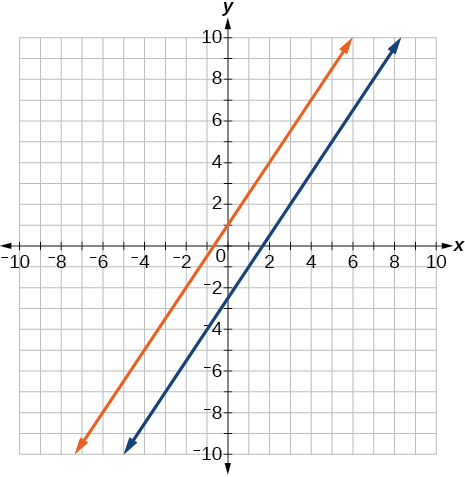Parallel [/hidden-answer] $\begin{array}{l}y=\frac{3x+1}{4}\hfill \\ y=3x+2\hfill \end{array}$ $\begin{array}{l}x=4\\ y=-3\end{array}$ [reveal-answer q=”fs-id1570411″]Show Solution[/reveal-answer] [hidden-answer a=”fs-id1570411″]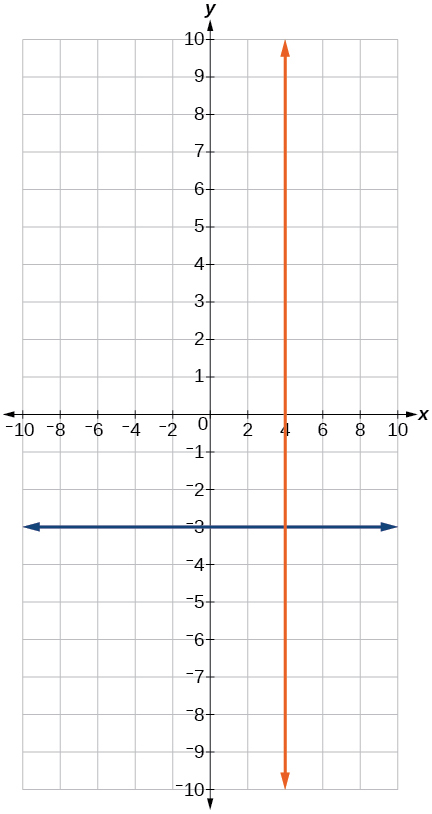Perpendicular [/hidden-answer] #### Numeric For the following exercises, find the slope of the line that passes through the given points. $\left(5,4\right)\,$and$\,\left(7,9\right)$ $\left(-3,2\right)\,$and$\,\left(4,-7\right)$ [reveal-answer q=”fs-id1595641″]Show Solution[/reveal-answer] [hidden-answer a=”fs-id1595641″] $m=\frac{-9}{7}$ [/hidden-answer] $\left(-5,4\right)\,$and$\,\left(2,4\right)$ $\left(-1,-2\right)\,$and$\,\left(3,4\right)$ [reveal-answer q=”fs-id1393112″]Show Solution[/reveal-answer] [hidden-answer a=”fs-id1393112″] $m=\frac{3}{2}$ [/hidden-answer] $\,\left(3,-2\right)$and$\,\left(3,-2\right)$ For the following exercises, find the slope of the lines that pass through each pair of points and determine whether the lines are parallel or perpendicular. $\begin{array}{l}\left(-1,3\right)\text{ and }\left(5,1\right)\\ \left(-2,3\right)\text{ and }\left(0,9\right)\end{array}$ [reveal-answer q=”fs-id1391026″]Show Solution[/reveal-answer] [hidden-answer a=”fs-id1391026″] ${m}_{1}=\frac{-1}{3},\text{ }{m}_{2}=3;\text{ }\text{Perpendicular}\text{.}$ [/hidden-answer] $\begin{array}{l}\left(2,5\right)\text{ and }\left(5,9\right)\\ \left(-1,-1\right)\text{ and }\left(2,3\right)\end{array}$ #### Technology For the following exercises, express the equations in slope intercept form (rounding each number to the thousandths place). Enter this into a graphing calculator as Y1, then adjust the ymin and ymax values for your window to include where the y-intercept occurs. State your ymin and ymax values. $0.537x-2.19y=100$ [reveal-answer q=”fs-id2716604″]Show Solution[/reveal-answer] [hidden-answer a=”fs-id2716604″] $y=0.245x-45.662.\,$Answers may vary.$\,{y}_{\text{min}}=-50,\text{ }{y}_{\text{max}}=-40$ [/hidden-answer] $4,500x-200y=9,528$ $\frac{200-30y}{x}=70$ [reveal-answer q=”fs-id2435252″]Show Solution[/reveal-answer] [hidden-answer a=”fs-id2435252″] $y=-2.333x+6.667.\,$Answers may vary.$\,{y}_{\mathrm{min}}=-10, {y}_{\mathrm{max}}=10$ [/hidden-answer] #### Extensions Starting with the point-slope formula$\,y-{y}_{1}=m\left(x-{x}_{1}\right),$solve this expression for$\,x\,$in terms of$\,{x}_{1},y,{y}_{1},$and$\,m.$ Starting with the standard form of an equation$\,\text{A}x\text{ + B}y\text{ = C,}$solve this expression for y in terms of$\,A,B,C,\,$and$\,x.\,$Then put the expression in slope-intercept form. [reveal-answer q=”fs-id2485835″]Show Solution[/reveal-answer] [hidden-answer a=”fs-id2485835″] $y=\frac{-A}{B}x+\frac{C}{B}$ [/hidden-answer] Use the above derived formula to put the following standard equation in slope intercept form:$\,7x-5y=25.$ Given that the following coordinates are the vertices of a rectangle, prove that this truly is a rectangle by showing the slopes of the sides that meet are perpendicular. $\left(-1,1\right),\left(2,0\right),\left(3,3\right)\text{,}$and$\,\left(0,4\right)$ [reveal-answer q=”fs-id1398905″]Show Solution[/reveal-answer] [hidden-answer a=”fs-id1398905″] $\begin{array}{l}\text{The slope for }\left(-1,1\right)\text{ to }\left(0,4\right)\text{ is }3.\\ \text{The slope for }\left(-1,1\right)\text{ to }\left(2,0\right)\text{ is }\frac{-1}{3}.\\ \text{The slope for }\left(2,0\right)\text{ to }\left(3,3\right)\text{ is }3.\\ \text{The slope for }\left(0,4\right)\text{ to }\left(3,3\right)\text{ is }\frac{-1}{3}.\end{array}$ Yes they are perpendicular. [/hidden-answer] Find the slopes of the diagonals in the previous exercise. Are they perpendicular? #### Real-World Applications The slope for a wheelchair ramp for a home has to be$\,\frac{1}{12}.\,$If the vertical distance from the ground to the door bottom is 2.5 ft, find the distance the ramp has to extend from the home in order to comply with the needed slope.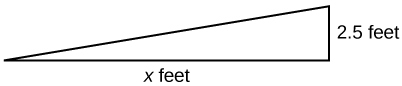[reveal-answer q=”fs-id2787116″]Show Solution[/reveal-answer] [hidden-answer a=”fs-id2787116″] 30 ft [/hidden-answer] If the profit equation for a small business selling$\,x\,$number of item one and$\,y\,$number of item two is$\,p=3x+4y,$find the$\,y\,$value when$\,p=\text{\}453\text{ and }x=75.$ For the following exercises, use this scenario: The cost of renting a car is$45/wk plus $0.25/mi traveled during that week. An equation to represent the cost would be$\,y=45+.25x,$where$\,x\,$is the number of miles traveled. What is your cost if you travel 50 mi? [reveal-answer q=”fs-id3148392″]Show Solution[/reveal-answer] [hidden-answer a=”fs-id3148392″]$57.50

If your cost were$\,\text{\}63.75,$how many miles were you charged for traveling?

Suppose you have a maximum of \$100 to spend for the car rental. What would be the maximum number of miles you could travel?

220 mi

### Glossary

conditional equation
an equation that is true for some values of the variable
identity equation
an equation that is true for all values of the variable
inconsistent equation
an equation producing a false result
linear equation
an algebraic equation in which each term is either a constant or the product of a constant and the first power of a variable
solution set
the set of all solutions to an equation
slope
the change in y-values over the change in x-values
rational equation
an equation consisting of a fraction of polynomials